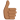# Is the derived formula valid?

• B
ForceBoy
Kinetic Energy is defined as the product of half the mass of an object multiplied by the square of its velocity. Using this I derived the end formula. Work is as follows:

KE = ½(m⋅v2)

Because v = vi +at, if vi = 0,

KE = ½(m⋅(at)2)

KE = ½(m⋅a2⋅t2)

KE = ½(m⋅a⋅a⋅t2)

Substituting F for m⋅a (force is equal to mass by acceleration),

KE = ½(F⋅a⋅t2)

Displacement is equivalent to vi⋅t +½⋅a⋅t2
It was stated that vi = 0, therefore s = ½⋅a⋅t2
Making this replacement in the equation yields:

KE = F⋅ s

Where F is a force and s is a displacement. This is also the formula for work. Then, is work the same thing as Kinetic Energy? Can the formula for work be used to calculate Kinetic Energy?

Homework Helper
Yes, that's often called the "Work-Energy Theorem". It's just conservation of energy. If you put work into the motion of an object, that object's kinetic energy increases by the amount of work you put in.

Your derivation used some formulas that assume straight line motion, constant force and constant acceleration. But it's a more general result than that. If you put energy into an object, the object's energy increases by that much.

That concept can be used in all sorts of situations. Suppose you throw a ball up in the air. How high will it go? Answer: It will rise until its initial kinetic energy (1/2)mv^2 has all been turned into work against gravity mg * h. So you set those two things equal and solve for h. KE = work.

If you have an object moving with velocity v and a frictional force F acts on it, how far does it slide before friction stops it? It takes until the work Fd done by friction is equal to the amount of energy (1/2)mv^2 that the object started with. Again, set KE = work.

•ForceBoy
ForceBoy
Thank you. Have a good day.